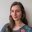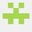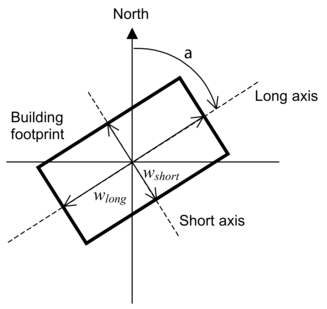Question-and-Answer Resource for the Building Energy Modeling Community
Get started with the Help page

# "Long Axis of Building" fields on Airflow Network: Simulation ControlSo, my question is short and "simple": for a rectangular building with longer South-North façades, in the 2 "Long Axis of Building" fields in the Airflow Network's Simulation Control, do I have to input 90º and below the ratio (not 1), or not? I ask this because I commonly see both of these left as default for rectangular buildings with larger S-N than E-W façades. And I do want it to calculate the Wind Pressure Coefficient. (I have GlobalGeometryRules as Relative, but the true North angle set as 0º in Building.)

edit retag close merge delete

Sort by » oldest newest most votedIf I understand your question correctly, you have set "Wind Pressure Coefficient Type" to SurfaceAverageCalculation and you are asking about the "Azimuth Angle of Long Axis of Building" and "Ratio of Building Width Along Short Axis to Width Along Long Axis" fields. Assuming that is correct, yes, you should input 90 and the ratio. Here's the picture that's in the docs:The calculation that is done here is not directly influenced by the geometry rules as far as I know. The code computes a wind pressure curve for each of the four cardinal direction (N, E, S, W) and then associates the surfaces (which have had geometry rules applied) to these directions. The closer that your building is to being a square, the less the angle and ratio will matter. I have also seen these left as the defaults, even with non-rectangular buildings, which isn't super great, but is probably an OK approximation in the absence of better data (e.g. computed from CFD).

more

Yes, it was precisely that that I was referring to. I had inserted both values just in case, but now I'm sure. Thank you very much for the enlightenment and kind reply!